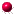XMM-Newton Science Analysis System

### Behaviours and command-line arguments.

This task accepts either an event or image file as an input (controlled by inputstyle), and outputs either the sky coordinate image or 3-dimensional chipcube (controlled by outputstyle).

In short, we recommend to specify an event file as an input, and the sky coordinate image as an output, of which the reasons are described below.

1. if the input is an event file and the output is the chipcube, the process runs as follows:
The task calculates the normalised count-per-frame-per-pixel (=rate) map for each chip, taking account of the energy (PI) of each event. Then the pixels, where the rate exceeds the given threshold, are masked and it is output.

2. if the input is an event file and the output is the sky-coordinate image, the process runs as follows:
The core of the routine is exactly the same as the above case. However, the coordinate conversion into sky-coordinates is not a trivial job, as the pile-up phenomenon is basically processed on the chip(raw) coordinates. Hence a user is allowed to specify the binned-attitude file. If this (binnedattset) is set, the task splits the event files into the given time bins (described in the file binnedattset), performs the above-described calculation (of the normalised rate map) for each of these time bins, converts each normalised rate map and mask map into the sky coordinates. Finally the task calculates the logical sum of the mask file. In other words, all the pixels that experience the rate above the threshold in one of the time bins are masked. Note that frames with too short duration are not used in this calculation of rate and mask images (Controlled by avcnttoprocess) in this case. If a user specifies so (controlled by attstyle), the entire observation is used as one set — in that case, the spatial fluctuation of the spacecraft is not taken into account (the median attitude value is simply used for the coordinate conversion).

3. if the input is an image file and the output is the chipcube,
the process is similar to the case 1. However the energy of each event is unknown. Therefore the image is assumed to have a single energy, which is the mean energy of those specified by the user (Controlled by evlo and evhi). As for the attitude of the spacecraft, which is used in converting the sky coordinates to the chip ones, the median attitude value is simply used.

A potential problem is when the image bin size is larger than the chip-coordinate one, the output mask on the chip-coordinates will be sparse, because only the central value of the image coordinates are taken into account in the current algorithm. For example, suppose the former is twice as larger than the latter, and all the image pixels exceed the piled-up threshold, then only one in every 4 pixels in the chipcube is flagged as piled-up.

4. if the input is an image file and the output is the sky-coordinate image, too,
the task does the same thing as the above case, although the output file is in the same coordinates. However, the spacecraft attitude information is still needed to get the (number of) frame information for each pixel. The median attitude value is used for that purpose.

Note the algorithm is identical to that in eootepileupmask. Consult its manual for detail.

XMM-Newton SOC -- 2023-04-16